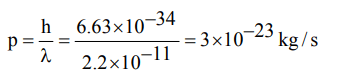## Register Now

If wavelength of photon is $2.2×10^{-11}m, h=6.6 ×10^{-34}Js$       , then momentum of photon is
a) $3 × 10^{-23}Kg/s$
b) $3.33× 10^{22}Kg/s$
c) $1.452× 10^{-44}Kg/s$
d) $6.89× 10^{43}Kg/s$